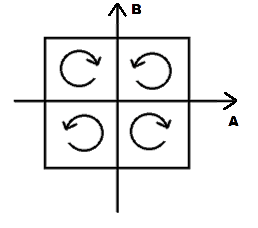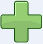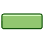# Geometric meaning of signs in multiplications

• B
• hatem240600
Multiplying by an Inverse: Example × two positives make a positive: 3 × -2 = 1...or two negatives make a negative: -3 × -2 = 1The proofs that units of a ring form a multiplicative group, and that a group has a unique neutral element and unique inverse elements depend a bit on how you define a group (laws vs. solvability of equations).f

#### hatem240600

What does 1/-1 (one divided by minus one) mean?
What does -1 X -1 (minus one multiplied by minus one) mean?
What are the best graphic representations of multiplication and division?

Welcome to PF!

What is the context of these questions?

Are you taking a course in Algebra?

Came across this cute little example, showing concepts of negatives and positives:

The enemy (-) of (*) my enemy (-) is my friend (+).
The friend (+) of (*) my enemy (-) is my enemy (-).
The enemy (-) of (*) my friend (+) is my enemy (-).
The friend (+) of (*) my friend (+) is my friend (+).

•hatem240600 and jedishrfu
What does 1/-1 (one divided by minus one) mean?
For starters, division is defined as the inverse of multiplication.

So, 4 x 2 = 8 has the inverse operation of 8 / 2 = 4.
And 4 x -2 = -8 has the inverse: -8 / -2 = 4.

What does -1 X -1 (minus one multiplied by minus one) mean?
Not the best example, but...

I dove to 60 feet below sea level (-60) in 10 intervals of 6 foot descents (10 x -6 = -60).

How far from my final depth was I when I was two descents away (i.e. 2 less than)?
-6 x -2 = 12
I was 12 feet from my final depth.

What are the best graphic representations of multiplication and division?
Often, debts and assets.

•hatem240600
OP, you just have to learn it. -1*-1 = +1. The minuses cancel. 1 / (-1) = -1 because there is only 1 minus. $${1 \over -1} = {-1 \over 1} = -{1 \over 1} = -1$$

•hatem240600
Actually you should write

##1/-1 = (1/-1)*(-1/-1) = (1*-1)/(-1*-1) = -1/1 = -1##

•hatem240600
What does 1/-1 (one divided by minus one) mean?
What does -1 X -1 (minus one multiplied by minus one) mean?
What are the best graphic representations of multiplication and division?
The best explanation I found was the following:

##1 \cdot 1 = 1## is the area of a square with side length ##1##. Now think of an orientation of this area. Then the signs lead toA purely algebraic answer would probably lead a bit far as a few definitions and concepts will be needed.

#### Attachments

•hatem240600, jedishrfu and suremarc
•hatem240600 and jedishrfu
Thank you all guys. Sorry for the late response. I really appreciate your help. I am just trying to "put a face" on a concept that seems vague for me.

•jedishrfu
Welcome to PF!

What is the context of these questions?

Are you taking a course in Algebra?
Just trying to understand!

For starters, division is defined as the inverse of multiplication.

So, 4 x 2 = 8 has the inverse operation of 8 / 2 = 4.
And 4 x -2 = -8 has the inverse: -8 / -2 = 4.

Not the best example, but...

I dove to 60 feet below sea level (-60) in 10 intervals of 6 foot descents (10 x -6 = -60).

How far from my final depth was I when I was two descents away (i.e. 2 less than)?
-6 x -2 = 12
I was 12 feet from my final depth.

Often, debts and assets.
Thank you! These are really good examples!

The best explanation I found was the following:

##1 \cdot 1 = 1## is the area of a square with side length ##1##. Now think of an orientation of this area. Then the signs lead to

View attachment 229859

A purely algebraic answer would probably lead a bit far as a few definitions and concepts will be needed.
Please share. I think I can handle some algebra.

•jedishrfu
•jedishrfu
Please share. I think I can handle some algebra.
##\{\,-1,+1\,\}## are the units in the ring of integers, which is the basic domain our number system with characteristic zero is built upon. The integers are the basis. In fact, it are the natural numbers and the integers are already the first step, in which we constructed a group from the monoid ##(\mathbb{N},+)## in order to reverse additions. As it turns out, ##\mathbb{Z}## is also a ring, i.e. allows a distributive multiplication. Now the units of any ring form a multiplicative group, in this case of order ##2##. There is only one neutral element in a group, which we usually write as ##1## in the multiplicative case (##0## in the additive case). This requires to have ##(-1)\cdot 1 = -1## from which all other formulas follow:

##(1 / (-1))= 1\cdot (-1)^{-1}=(-1)^{-1}= -1## for otherwise we would have
##-1\stackrel{(*)}{=}1\cdot (-1)\stackrel{(**)}{=}(-1)^{-1}\cdot (-1)\stackrel{(***)}{=}1## which cannot be in the case of characteristic zero.
##(*)## definition of unique ##1##
##(**)## assumption ##(-1)^{-1}= 1##
##(***)## definition of unique inverse

Next we have ##(-1)\cdot (-1)\stackrel{(a)}{=}(-1)\cdot (-1)^{-1}\stackrel{(b)}{=}1##
##(a)## by the previous formula
##(b)## by the definition of the inverse

The proofs that units of a ring form a multiplicative group, and that a group has a unique neutral element and unique inverse elements depend a bit on how you define a group (laws vs. solvability of equations).

When We Multiply:
Example×two positives make a positive:3 × 2 = 6×two negatives make a positive:(−3) × (−2) = 6×a negative and a positive
make a negative:(−3) × 2 = −6×a positive and a negative
make a negative:3 × (−2) = −6
Yes indeed, two negatives make a positive, and we will explain why, with examples!

Signs

"+" is the positive sign, "−" is the negative sign.

When a number has no sign it usually means that it is positive.

Example: 5 is really +5

#### Attachments

I don't think the OP is asking about the mechanics of computing the result of a multiplication.

A multiplication of two positive numbers leads to the direct and intuitively obvious rectangle. One can put the rectangle on its side and immediately see that X * Y is the same as Y * X. I thought this was very cool.

Negative numbers are a bit harder to grasp geometrically. One can talk about holes, I suppose, but a hole needs depth, and a rectangle has no depth. Erase a rectangle out of a larger area, I guess?

Negative numbers are a bit harder to grasp geometrically. One can talk about holes, I suppose, but a hole needs depth, and a rectangle has no depth. Erase a rectangle out of a larger area, I guess?
The clue lies in the insight, that volume is an orientated quantity!
This explains ##(-1)\cdot (-1) = 1## up to ##v \wedge w = - w \wedge v##.

Strangely this fact isn't questioned in case the volume is a length. Measured to the left differs from measured to the right and nobody asks why. But this observation doesn't change with dimension. That's all.

What does 1/-1 (one divided by minus one) mean?
What does -1 X -1 (minus one multiplied by minus one) mean?
What are the best graphic representations of multiplication and division?
Number Line; the real number line, using zero as a reference point, negative numbers pointing to the left and positive numbers pointing to the right. Start is to learn addition and subtraction on the number line, as done in first grade, and as done again when beginning to learn algebra. From this, Multiplication is reviewed and is also given the reminder that multiplication is a shortened way of representing Addition.

The posts which came before this one were also helpful. Those are discussing the rules.